Documentation

Simulink® is a graphical modeling and simulation environment for dynamic systems. You can create block diagrams, where blocks represent parts of a system. A block can represent a physical component, a small system, or a function. An input/output relationship fully characterizes a block. Consider these examples:

• A faucet fills a bucket — Water goes into the bucket at a certain flow rate, and the bucket gets heavier. A block can represent the bucket, with flow rate as the input and its weight as the output.

• You use a megaphone to make your voice heard — The sound produced at one end of the megaphone is amplified at the other end. The megaphone is the block, the input is the sound wave at its source, and the output is the sound wave as you hear it.

• You push a cart and it moves — The cart is the block, the force you apply is the input, and the cart's position is the output.

The definition of a block is only complete with its inputs and outputs defined; this task relates to the goal of the model. For example, the cart velocity may be a natural choice as an output if the modeling goal does not involve its location.

Simulink provides block libraries that are collections of blocks grouped by functionality. For example, to model a megaphone that multiplies its input by a constant, you use a Gain block from the Math Operations library.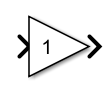A sound wave goes into the megaphone as its input, and a louder version of the same wave comes out as its output.

The > signs denote the inputs and outputs of a block, which can be connected to other blocks.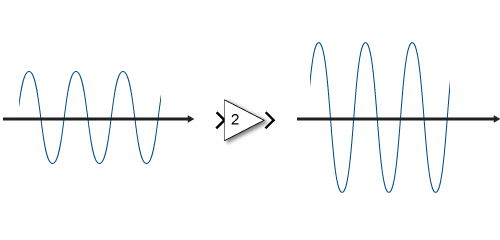You can connect blocks to other blocks to form systems and represent more complex functionality. For example, an audio player turns a digital file into sound. A digital representation is read from storage, is interpreted mathematically, and then turned into physical sound. The software that processes the digital file to compute the sound waveform can be one block; the speaker that takes the waveform and turns it into sound can be another block. A component that generates the input is another block.

To model the sine wave input to the megaphone in Simulink, include a Sine Wave source.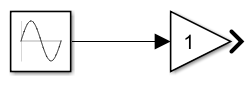The primary function of Simulink is to simulate behavior of system components over time. In its simplest form, this task involves keeping a clock, determining the order in which the blocks are to be simulated, and propagating the outputs computed in the block diagram to the next block. Consider the megaphone. At each time step, Simulink must compute the value of the sine wave, propagate it to the megaphone, and then compute the value of its output.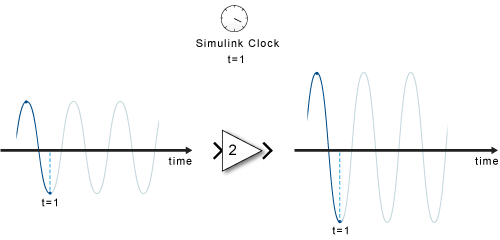At each time step, each block computes its outputs from its inputs. Once all of the signals in a diagram are computed at a given time step, Simulink determines the next time step (based on the model configuration and numerical solver algorithms) and advances the simulation clock. Then each block computes their output for this new time step.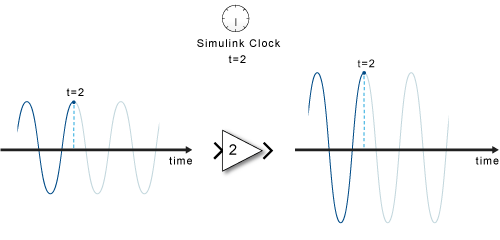In simulation, time progresses differently from a real clock. Each time step takes as much time as it takes to finish the computations for that time step, whether that time step represents a fraction of a second or a few years.

Often, the effect of a component's input on its output is not instantaneous. For example, turning on a heater does not result in an instant change in temperature. Rather, this action provides input to a differential equation. The history of the temperature (a state) is also a factor. When simulation requires solving a differential or difference equation, Simulink employs memory and numerical solvers to compute the state values for the time step.

Simulink handles data in three categories:

• Signals — Block inputs and outputs, computed during simulation

• States — Internal values, representing the dynamics of the block, computed during simulation

• Parameters — Values that affect the behavior of a block, controlled by the user

At each time step, Simulink computes new values for signals and states. By contrast, you specify parameters when you build the model and can occasionally change them while simulation is running.